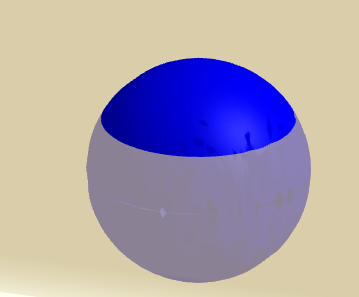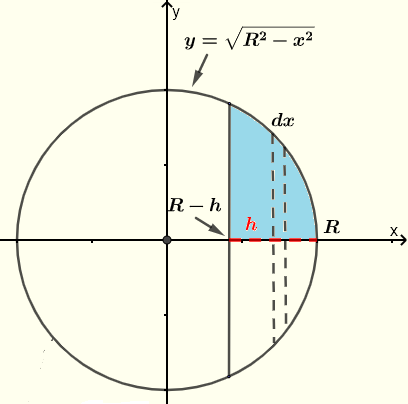# Volume of a Spherical Cap

   

The volume of a spherical cap is found using integrals and the method of disks used in "volume of a Solid of revolution".
A spherical cap is defined as as a portion of the sphere cut by a plane.We now consider a spherical cap from a sphere of radius R and height $h$. A spherical cap may be generated by revolving the curve of $y = \sqrt{R^2 - x^2}$, which is half a circle, around the x-axis with $x$ in the range $R-h \le x \le R$.Consider the small disk (in broken lines) having a width $dx$ and a radius equal to $y$. The volume of the disk is given by $\pi y^2 dx$ and thefore the integral over $x$ in the ramge $[R-h, R ]$ gives the volume of the cap.
$\displaystyle \text{Volume} = \int_{R-h}^{R} \pi y^2 dx$
Since $y = \sqrt{R^2 - x^2}$, the volume is given by
$\displaystyle \text{Volume} = \pi \int_{R-h}^{R} (R^2 - x^2) dx$
Evaluate the integral
$\displaystyle \text{Volume} = \pi \left[R^2 x - \dfrac{1}{3}x^3\right]_{R-h}^{R}$
Evaluate the above expression on the right
$\displaystyle \text{Volume} = \pi \left\{ \left(R^3 - \dfrac{1}{3}R^3\right) - \left(R^2 (R-h) - \dfrac{1}{3}(R-h)^3\right) \right\}$
Simplify the above to obtain the volume
$\Large \displaystyle \color{red} {\text{Volume} = \dfrac{\pi}{3}( 3 Rh^2-h^3) }$